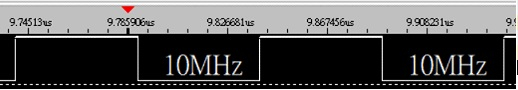# Setting the PWM frequency using the SDK

edited June 2018

In the SDK there appears to be only two commands in relation to the PWM, Set and get.

The "EApiPwmSetValue" uses 4 input values. But none of these values change the frequency of the PWM.

At present the minimum frequency at 17Mhz when the command is initialised with the following:
EApiPwmSetValue(0,1,0,1)
with the first variable being the PWM ID number,
Second is the Base Unit Integer
the third is the Base Unit fraction - which cannot be set to anything but 0 for some reason
the forth is the duty.

So the question is. How do you set the Upboard PWM frequency to its minimum of 293Hz?

Tagged:

• Hi rhulme77,
The minimum speed of PWM is 75kHz, so you can't set the PWM freq. to 293Hz. And the speed of signal frequency is changed from 75kHz ~ 10 MHz ~ 75kHz, is equal to 0x01 ~ 0xFF from Base Unit.
e.g. if set the Base Unit to 0x7F, the output frequency will be 10MHz, as shown in the picture below<-- by logic analyzer

• so why does the wiki state the following?

2 PWM ports, supporting frequencies between 293 Hz and 75 kHz with 8-bit duty-cycle resolution, are provided on the I/O header.

• edited July 2018

Please note, the PWM frequency of 293Hz should work on Linux for UP, but not for Windwos SDK